# 光学玻璃色散的应用

## 关于色散

$$V_D=\frac{n_D-1}{n_F-n_C}$$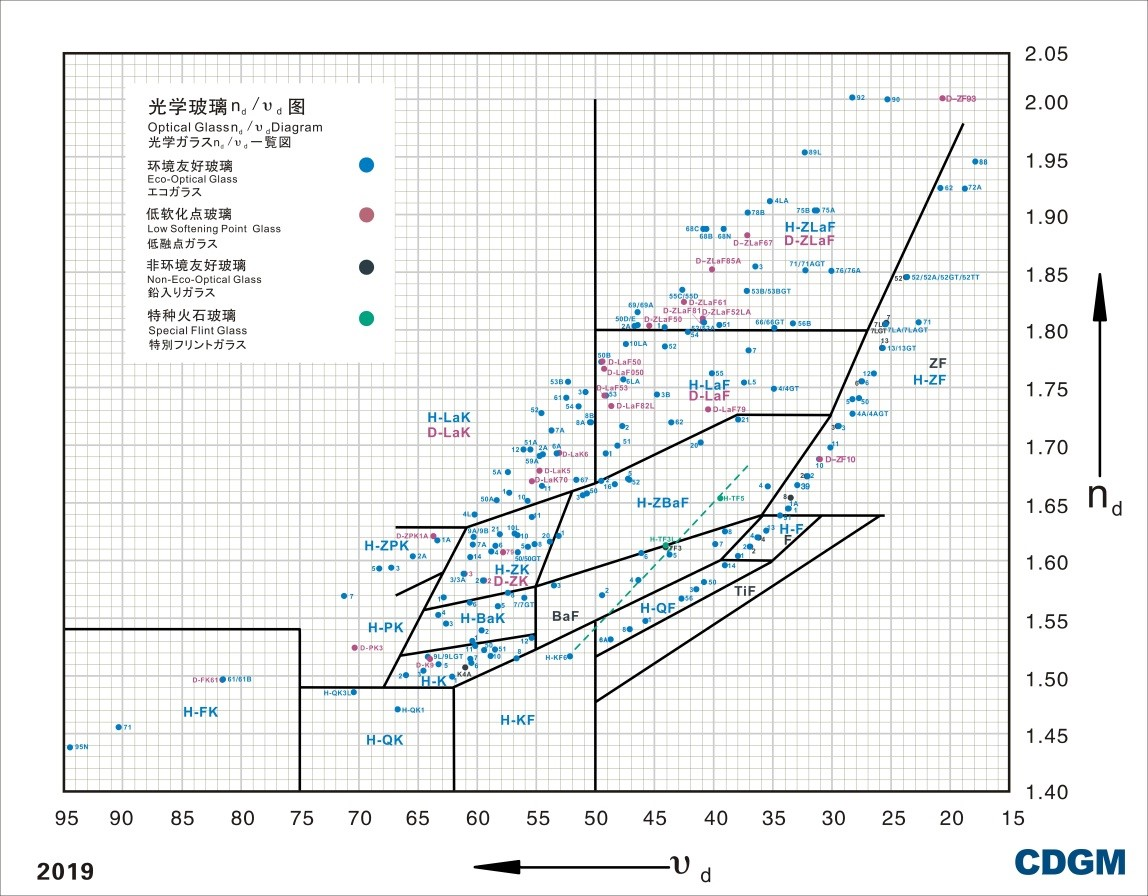◎ 图 1：成都光明公司 nD-vD 图◎ 图 2：3 种透明光学玻璃的色散曲线

$$n^2(\lambda)=A_0+A_1\lambda^2+A_2\lambda^{-2}+A_3\lambda^{-4}+A_4\lambda^{-6}+A_5\lambda^{-8}$$

## 折射率的测量方法

### V 棱镜法

V 棱镜是由两块材料完全相同并已知折射率为 $n_0$ 的直角等腰棱镜胶合而成，经细磨为 $90$ 度的待测玻璃试样放入 V 形缺口中，加浸液后可以进行测量。当一束单色平行光垂直入射 V 棱镜后，若被测试样的折射率与 V 棱镜折射率相同，光线将不偏折地通过 V 棱镜；若被测试样折射率 $n$ 与 V 棱镜折射率 $n_0$ 有差别，光线将遵循折射定律发生偏折（图 3）。设出射光线与入射光线的夹角为 $\theta$ 时，可以推导出 $\theta$ 与 $n$ 之间的关系为：

$$n=\sqrt{n_0^2\pm\sin\theta\sqrt{n_0^2-\sin^2\theta}}$$

$n>n_0$ 时，$\theta$ 为正数值；反之为负数值。所以只要测出偏折角 $\theta$ ，即可计算被测玻璃的折射率。◎ 图 3：V 棱镜法原理图

### 全反射法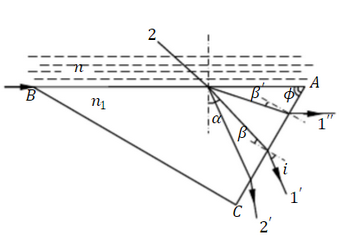◎ 图 4：全反射法原理图

### 最小偏向角法◎ 图 5：三棱镜偏向角示意图

$$\delta=(i_1-i_2)+(i_4-i_3)\tag{1}$$
$$A+\delta=i_1-i_4\tag{2}$$

$$\sin(\frac{A+\delta}{2})=n\sin\frac{\delta}{2}\cdot\frac{\cos\frac{i_2+i_3}{2}}{\cos\frac{i_1+i_2}{2}}\tag{3}$$

$(3)$ 式说明，当顶角 $A$ 和折射率 $n$ 已定，偏向角 $\delta$ 是 $i_1$ 的函数。

$$\frac{d\delta}{di_1}=1-\frac{di_4}{di_1}\tag{4}$$

$$\frac{\cos{i_1}}{cos{i_2}}=\frac{\cos{i_4}}{cos{i_3}}\tag{5}$$

## 分光计的使用

Tip◎ 图 6：分光计示意图

• 螺钉 1：使望远镜和平行光管在子午面（图面）内作微小转动，使两光轴在子午内重合；
• 螺钉 2：望远镜和平行光管的光轴水平倾角调整螺钉；
• 螺钉 3：望远镜转角微调螺钉；
• 螺钉 4：望远镜锁紧螺钉；
• 螺钉 5：载物台下方的倾角调整螺钉；
• 螺钉 6：载物台锁紧螺钉；
• 螺钉 7：游标盘微调螺钉；
• 螺钉 8：游标盘锁紧螺钉；
• 螺钉 9：狭缝宽度调整螺钉。

### 仪器校准

Tip

#### 望远镜光轴与载物台转轴的校正

##### 平行平板的放置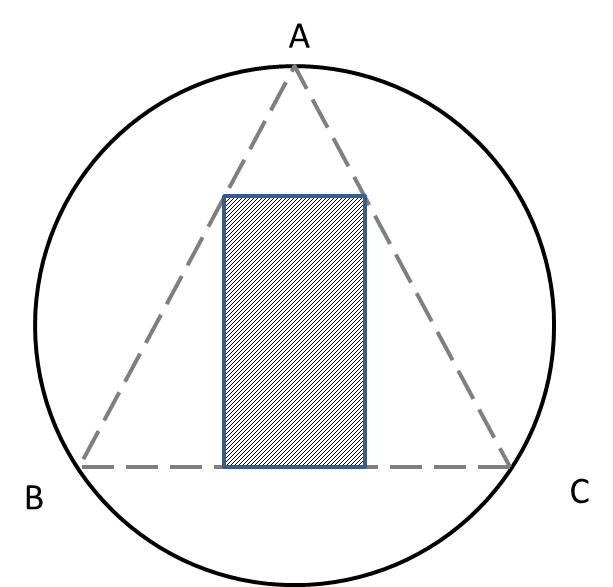◎ 图 7：平行平板放置方法示意图

##### 调整望远镜光轴使其与度盘转轴正交

$a$ 面调好后，载物台转 $180$ 度用同样的办法调整 $b$ 面，直至两面的反射像均位于上叉丝。再将平行平板旋转 $90$ 度，用同样的方法调整望远镜及载物台倾角使得无论平行平板哪一个面正对望远镜，反射的叉丝像均与原物对称。此时，望远镜瞄准轴与度盘旋转轴已垂直。◎ 图 8：1/2 修正法示意图

##### 平行光管的校准◎ 图 9：平行光管校准效果示意图

##### 调整棱镜抛光面法线与望远镜光轴平行◎ 图 10：三棱镜对称放置示意图

### 分光计读数◎ 图 11：分光计读数

$$N = \frac{A+B-180^{\circ}}{2}$$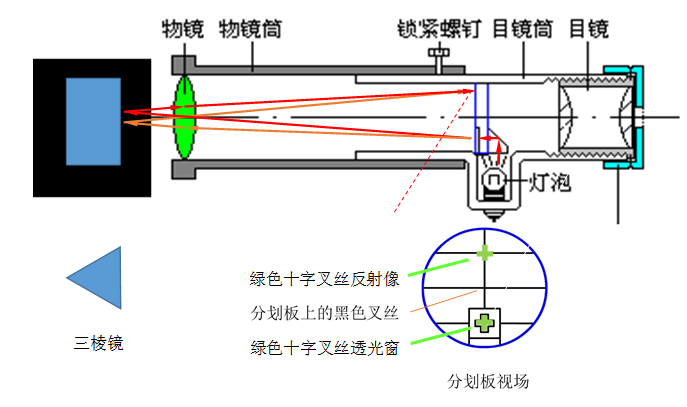◎ 图 12：自准直法

## 三棱镜顶角的测量方法

### 自准直法◎ 图 13：自准直法测顶角

### 反射法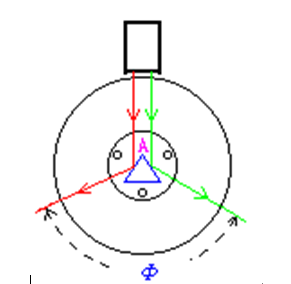◎ 图 14：反射法测顶角

## 玻璃折射率的测量

### 光源介绍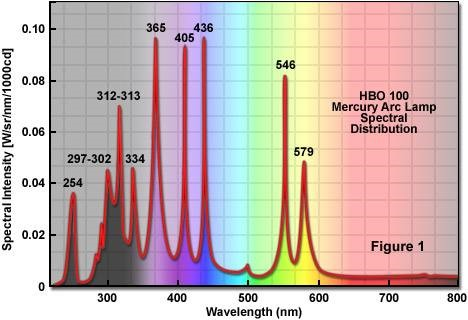◎ 图 15：波谱

### 测量方法

#### 单侧读数计算最小偏角◎ 图 16：单侧读数示意图

#### 双侧读数计算最小偏角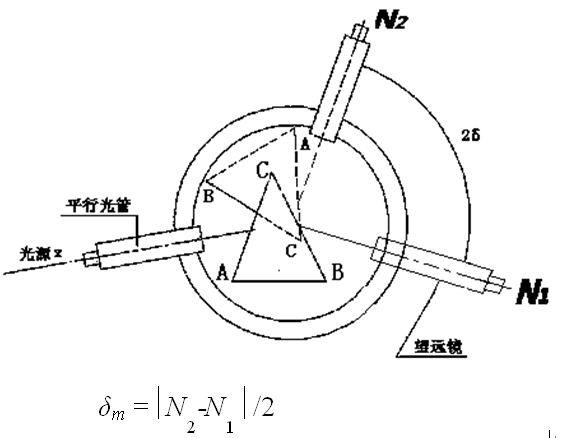◎ 图 17：双侧读数示意图

1. $1/2$ 修正法：当平行平板 $a$ 面对准望远镜时，观察反射像与上叉丝的偏移量 $h$，调节望远镜的下方的螺钉 1，使反射像上升（下降）$h/2$；再调整载物台下方螺钉 $B$（图 7）使反射像恰在上叉丝（图 8）。

2. 低压 Hg 灯的橙色谱线，实际为双线结构，一条 $576.96nm$，一条 $579.06nm$，因为谱线非常靠近，眼睛无法分辨时可视为一条谱线，取波长 $579nm$。